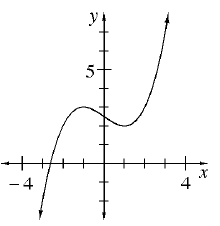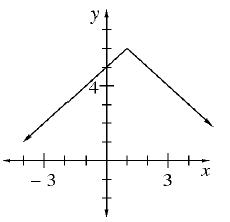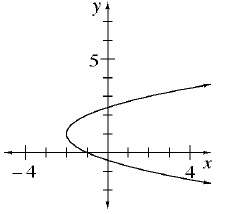### Home > CCA > Chapter 2 > Lesson 2.2.1 > Problem2-52

2-52.

Which graphs below have a domain of all numbers? Which have a range of all numbers? Which are functions?

1.1.1.In which graph do all values of $x$ have a corresponding $y$-value?
Refer to the Math Notes box in Lesson 1.2.5.

Graphs (a) and (b)

In which graphs do all values of $y$ have a corresponding $x$-value?

Graphs (a) and (c)

A function can only have one $y$-value for each $x$-value.